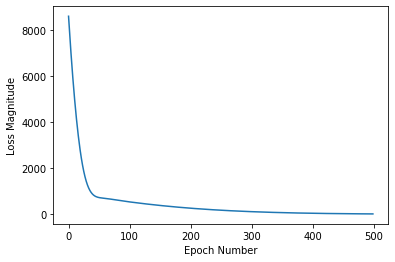# 把视角拉高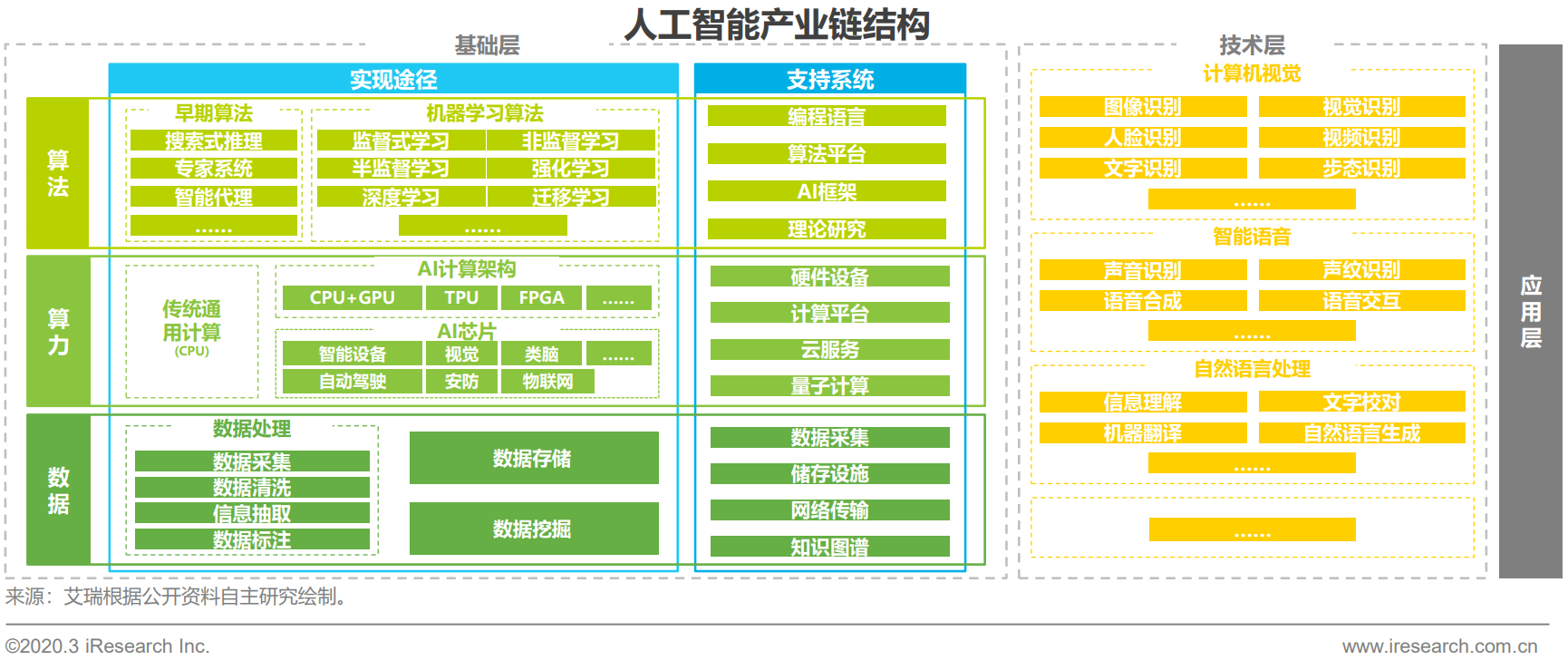• 监督学习：数据样本有标签。
• 非监督学习：数据样本无标签。
• 半监督学习：数据样本有部分(少量)标签。
• 强化学习：趋向结果则奖励，偏离结果则惩罚。

# 机器学习工业化流程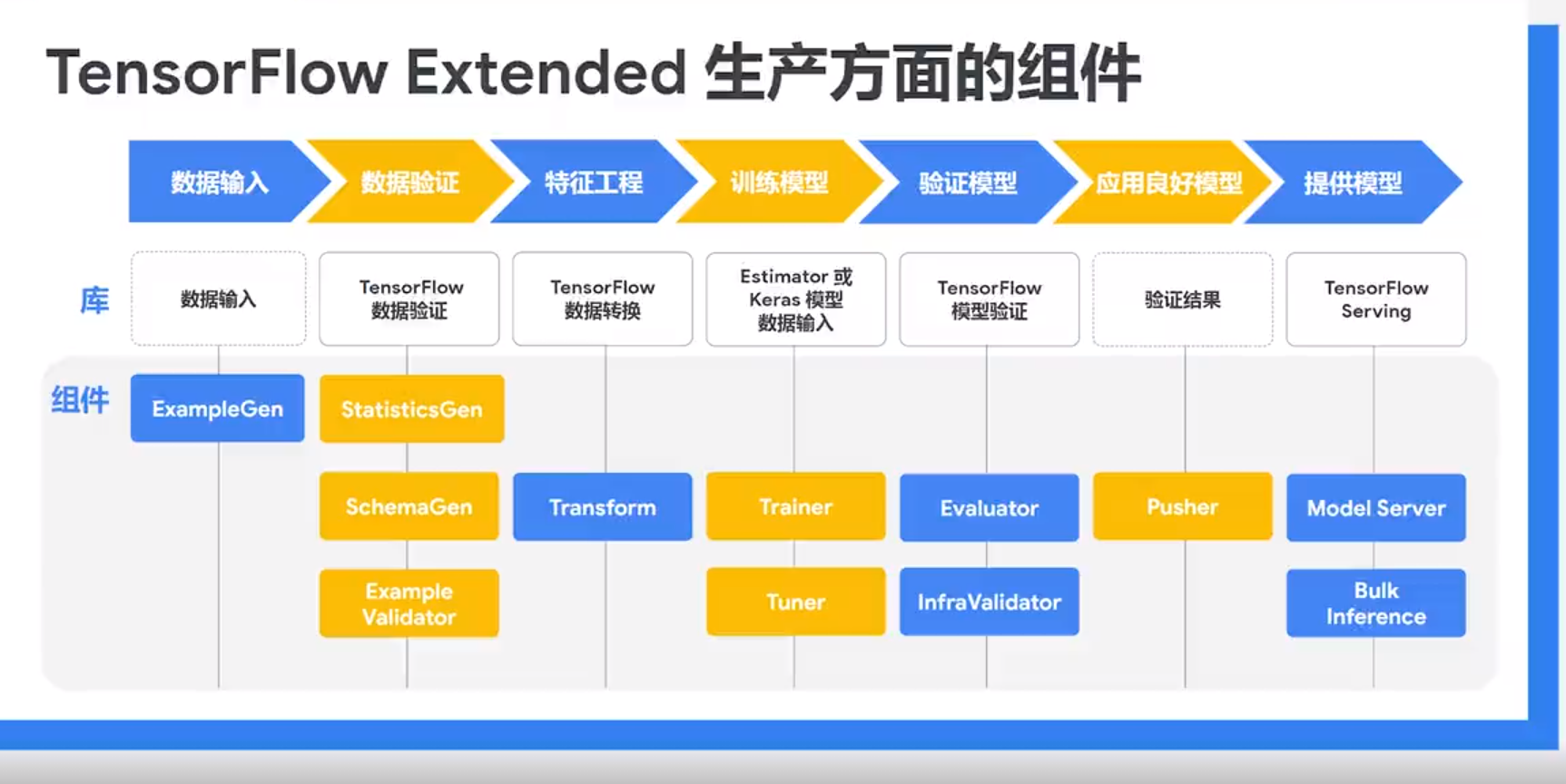1. 输入数据，并根据需要拆分数据集。
2. 生成训练数据和服务数据的特征统计信息。通过从训练数据中推断出类型、类别和范围来创建架构。识别训练数据和服务数据中的异常值。
3. 对数据集执行特征工程。
4. 训练模型，调整模型的超参数。
5. 对训练结果进行深入分析，并帮助验证导出的模型。检查模型是否确实可以从基础架构提供服务，并防止推送不良模型。
6. 将模型部署到服务基础架构。

# 机器是如何学习的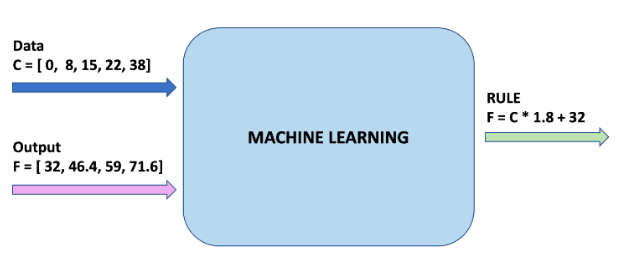• 特征：我们模型的输入。 在这种情况下，只有一个值-摄氏度。
• 标签：我们的模型预测的输出。 在这种情况下，只有一个值-华氏度。
• 实例：训练期间使用的一对输入/输出。 在我们的例子中，是摄氏度/华氏度一对数据，例如，(0, 32), (8, 46.4)。

• 拟合：通过训练数据，使模型来概括表示数据的过程。
• 模型：图结构，包含了训练过程中的权重与偏差的数据。其中的图为由各函数组成的计算结构。

# 简单上手机器学习代码

import numpy as np
import matplotlib.pyplot as plt
celsius    = [[-40], [-10], [ 0], [ 8], , , [ 38]]
fahrenheit = [[-40], [ 14], , [46.4], , [71.6], [100.4]]
plt.scatter(celsius,fahrenheit, c='red', label='real')
plt.xlabel('celsius')
plt.ylabel('fahrenheit')
plt.legend()
plt.grid(True)
plt.title('real data')
plt.show()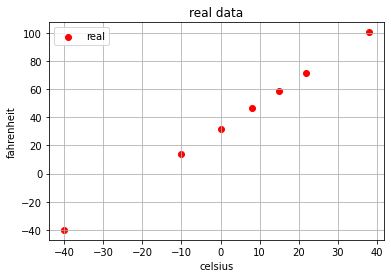from sklearn.linear_model import LinearRegression
lr = LinearRegression()
lr.fit(celsius,fahrenheit)


celsius_test = [[-50],[-30],,,,]
fahrenheit_test = lr.predict(celsius_test)
plt.scatter(celsius,fahrenheit, c='red', label='real')
plt.scatter(celsius_test,fahrenheit_test, c='orange', label='predicted')
plt.xlabel('celsius')
plt.ylabel('fahrenheit')
plt.legend()
plt.grid(True)
plt.title('estimated vs real data')
plt.show()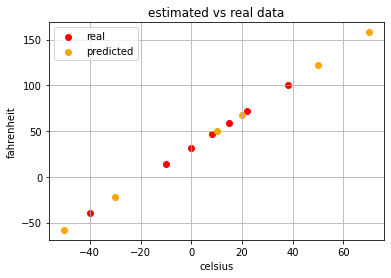# 顺带一提的深度学习代码

import tensorflow as tf
import numpy as np

# prepare data
celsius_q    = np.array([-40, -10,  0,  8, 15, 22,  38],  dtype=float)
fahrenheit_a = np.array([-40,  14, 32, 46.4, 59, 71.6, 100.4],  dtype=float)

# fit model
model = tf.keras.Sequential([tf.keras.layers.Dense(units=1, input_shape=)])
history = model.fit(celsius_q, fahrenheit_a, epochs=500, verbose=False)
print("Finished training the model")

# print loss
import matplotlib.pyplot as plt
plt.xlabel('Epoch Number')
plt.ylabel("Loss Magnitude")
plt.plot(history.history['loss'])